# Common Core HS Algebra: Quadratics Chapter Exam

Exam Instructions:

Choose your answers to the questions and click 'Next' to see the next set of questions. You can skip questions if you would like and come back to them later with the yellow "Go To First Skipped Question" button. When you have completed the practice exam, a green submit button will appear. Click it to see your results. Good luck!

### Page 2

#### Question 9 9. Solve the equation below.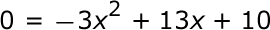### Page 3

#### Question 11 11. Solve the equation below.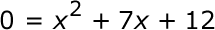#### Question 12 12. If the equation below told you a ball was 'h' feet in the air after 't' seconds, what would be the first step to finding out when it hit the ground?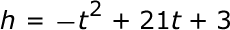#### Question 15 15. Find the value for c and rewrite the equation in the vertex form.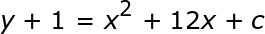### Page 4

#### Question 17 17. In order to complete the square on the following equation, what value would you add to both sides?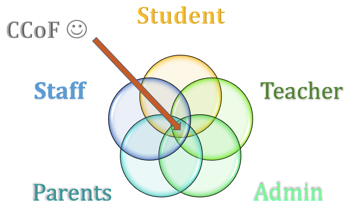#### Question 19 19. Rewrite the quadratic below in standard form: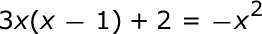### Page 5

#### Question 24 24. What would the first step be in completing the square of the following equation?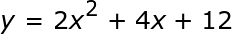#### Question 25 25. Expressing the equation below in the vertex form will give a value of 'h' = _____ .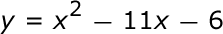### Page 6

#### Question 26 26. Find the roots of the equation below.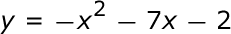#### Question 28 28. Solve the equation.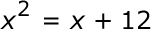#### Common Core HS Algebra: Quadratics Chapter Exam Instructions

Choose your answers to the questions and click 'Next' to see the next set of questions. You can skip questions if you would like and come back to them later with the yellow "Go To First Skipped Question" button. When you have completed the practice exam, a green submit button will appear. Click it to see your results. Good luck!

Support